# System of equations - 6th grade (11y) - math problems

#### Number of problems found: 64

• Have solutionThe sum of four consecutive even numbers is 92. Determine these numbers.
• BenchesThe park has 64 benches. Occupied are by 18 more than empty. How many benches are occupied and empty ?
• Purchase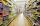Three buyers pay € 468. The first paid 3-times more than second, third half over second. How many euros paid each of them?
• WineA bottle of wine costs 21 euros; and wine is 20 times more expensive than a bottle. How much a bottle cost?
• Father and son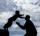Father and son are together 78 years. Son is 25 years younger than father. How old is the son?
• HostelStudents are accommodated in 22 rooms. Rooms were 4 and 6 bed. How many rooms in which type occupied 106 children there?
• Coffee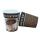Coffee from the machine in the cup cost 28 cents. Coffee is 20 cents more expensive than the cup. How much is the cup?
• FamilySon is 3/5 smaller than the mother. Mother is 99 cm higher than the son. How tall is the mother?The fruit basket is five times heavier than the empty basket. Filled is 20kg heavier than empty. Determine the weight of the fruit in the basket.
• RectangleThe length of one side of the rectangle is three times the length of the second side. What are the dimensions of the rectangle if its circumference 96 cm?
• Brothers and sistersLenka has as many brothers as sisters. Her brothers have twice as many sisters as brothers. How many brothers and sisters are in the family?
• Ratio three numbers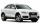Three numbers SUV are in the ratio 1:2:3. Their sum is 24. Find this numbers and write their add and sum.
• Pets in ZOOThe zoo have run for pets. They are chickens, pigs and cow. Altogether there has 20 heads and 60 feet. How many chickens and piglets are there?
• Rabbit family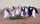Rabbit family ate 32 pieces of carrots, small one ate 6 pieces, dad 5 more than the mother. How much ate mom?
• Playing Cards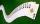Kara has 2 times more cards than Dana, Dana has 4× less than Mary. Together they have 728 cards. How many cards has each of them?
• Book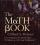John read the book so that it read 30 pages per day. Maxim read 34 pages a day and read it two days earlier than John. How many pages has the book?
• Chicken and rabbits (classic)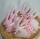Grandmother breeding chickens and rabbits. It found that the yard has 35 heads and 108 legs. How many chickens and rabbits breeding in the yard?
• Birds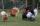On the farm they have a total of 110 birds. Geese and turkeys together is 47. Hens is three times more than the turkey. How much is poultry by species?
• GardensThe garden has the square shape with circumference 124 m. Divide it into two rectangular gardens, one should circumference 10 meters more than the second. What size will have a gardens?
• Cents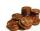Julka has 3 cents more than Hugo. Together they have 27 cents. How many cents has Julka and how many Hugo?

Do you have an interesting mathematical word problem that you can't solve it? Submit a math problem, and we can try to solve it.

We will send a solution to your e-mail address. Solved examples are also published here. Please enter the e-mail correctly and check whether you don't have a full mailbox.

Please do not submit problems from current active competitions such as Mathematical Olympiad, correspondence seminars etc...

Do you have a system of equations and looking for calculator system of linear equations? System of equations - math word problems. Examples for 6th grade (the sixth graders).